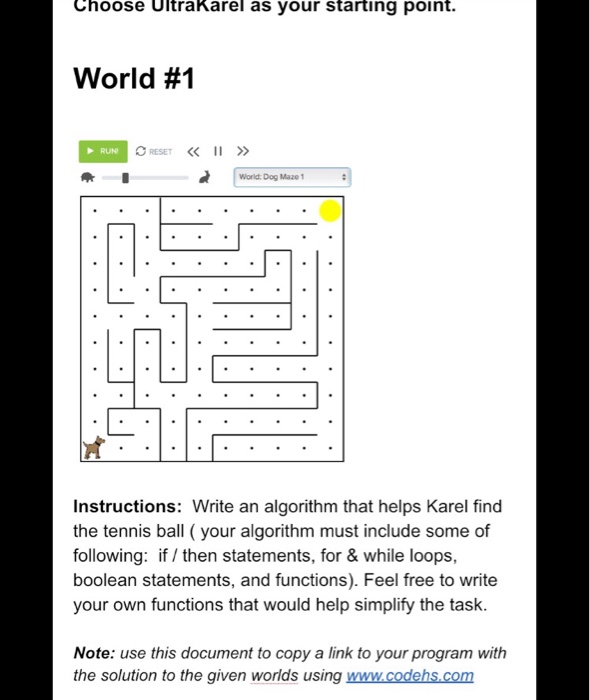# please help with the code that would solve this Choose Ultrakarel as your starting point. World #1 RESET II » RUN Wo...please help with the code that would solve this
Choose Ultrakarel as your starting point. World #1 RESET II » RUN World: Dog Maze1 Instructions: Write an algorithm that helps Karel find the tennis ball (your algorithm must include some of following: if / then statements, for & while loops, boolean statements, and functions). Feel free to write your own functions that would help simplify the task. Note: use this document to copy a link to your program with the solution to the given worlds using www.codehs.com

There are many ways to solve a maze, here we are going to consider the maze as a 2 dimensional array of values.

in our maze we can consider it as a 10x10 matrix.. (see the no. of dots vertically and horizontally).. let us consider 10 as the height of the maze and the other 10 as the width of the maze. now for each position we will assign a value. By doing that we will come to know the starting point the ending point,the ways that are blocked by walls,and the route taken.

so, it can be explained in simple steps:

step 1: enter the starting position.(in our case it is 0x0)

step 2: enter the end position..(in our case it is 10X10)

step 3: the row will increment , if the current position is not on a wall, the function will check other possible values for further movement,if such a value is not already visited it will follow that path, else if it is visited it will backtrack,visited positions are stored

step 4: if the current position is on the wall,then also it will do backtracking,

step 5: all above steps are repeated till it reaches the end position.

Algorithm:

int dog_maze[][] = new dog_maze[w][h];//we enter the maze in matrix

int x1,y1 //starting position

int x2,y2// end position

boolean[][] already_visited=boolean[w][h];// as i said already there will be a visited set

boolean[][] solution=boolean[w][h]// after reaching end position path is saved.

void help_dog()//function for solving the puzzle

{

dog_maze = createmaze()

for(row 0 to length)

for(column 0 to length)

solution[row][coloumn]=false;

}

public boolean tracking(int a, int b)

{

if(a==x2&&b==y2)//end position

return true;

return false;

if(a!=0)

if(help_dog(a-1,b))

{

solution[a][b]=true;

return true;

}

if(a!=w-1)

if(help_dog(a+1,b))

{

help_dog[a][b]=true;

return true;

}

if(b!=0)

if(help_dog(a,b-1))

{

solution[a][b]=true

}
}

now this function gets repeated till the end node is visited...

More Homework Help Questions Additional questions in this topic.